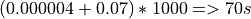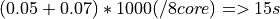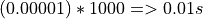# Wrapper development¶

## Pure python wrappers¶

Python wrappers aim to be an easy way for wrapping external code. The external code can be a mathematical formula or a coupling involving several computational codes dedicated to the resolution of a very complex physical problem.

Python wrappers are not the best solution if your external code last less than a microseconds and if you need to resolve billions of points. In that case, for better performance, consider using a compiled wrapper. In any other cases, a Python wrapper is the recommended choice.

### PythonFunction¶

The `PythonFunction` is a `Function` which execution is done in a Python context.

Here is an example of how to use it:

```import openturns as ot

def compute_point(X):
E, F = X
Y = E * F
return [Y]

model = ot.PythonFunction(2, 1, compute_point)
out_sample = model([[2, 3], [5, 8]])
```

Some explanations of the code :

• The `compute_point` function constructs the out point from the in point. The in and out array size must correspond to the sizes set in the PythonFunction constructor. In this example, X will be an array of size 2 and Y must be an array of size 1. The output point can be a Python list, an  Point or a Numpy array.

The evaluation can be done on several points to benefit from vectorization. It receives an input sample and must return an output sample. Here is an example:

```import openturns as ot
import numpy as np

def exec_sample(Xs):
# speedup using numpy
XsT = np.array(Xs).T
return np.atleast_2d(np.multiply(XsT, XsT)).T

model = ot.PythonFunction(2, 1, func_sample=exec_sample)
```

The output sample can be a Sample, a Python list of list or a Numpy array of array. This argument is optional. If `func_sample` is not provided and must compute a sample, the `func` function is called several time: once for each point of the sample. On contrary, if only `func_sample` is given and a point must be computed, the point is inserted in a sample of size 1, and extracted from the result sample.

The PythonFunction is quite simple to use. When used with coupling_tools module, it can wrap external program easily too.

### External code coupling tools¶

#### Simple example¶

Here is a simple example of wrapper where compute is made in an external program with the help of openturns.coupling_tools module:

```import openturns as ot
import openturns.coupling_tools as ct

external_program = 'python external_program.py'

def exec(X):
# create input file
in_file = 'input.py'
ct.replace('input_template.py', in_file, ['@E', '@F'], X)

# compute
ct.execute(external_program + ' ' + in_file)

# parse output file
Y = ct.get('output.py', tokens=['Z='])

return Y

model = ot.PythonFunction(2, 1, exec)
out_sample = model([[2, 3], [5, 8]])
print(out_sample)
```

Some explanations of the code :

• `replace()` replace `@E` and `@F` occurrence found in `input_template.py` file and write the result to `infile` file. `X` value will replace `'@E'` token and `X` will replace `'@F'` token.

• The external program is launched with `execute()`. The input filename is passed by parameters to the program.

• `get()` get the value following `'Z='` token in `output.py` file.

Template file example `input_template.py`:

```E=@E
F=@F
```

External program example `external_program.py`:

```#!/usr/bin/env python

# get input
import sys
inFile = sys.argv

# compute
Z = F * E

# write output
h = open('output.py', 'w')
h.write('Z=' + str(Z))
h.close
```

Output file example `output.py`:

```Z=42.0
```

#### More examples¶

The replace `replace()` function can edit file in place. It can format values in anyway. Actually, values can be of type “string”, if not, they are converted using str() Python function:

```replace(outfile='input_template.py', infile=None,
tokens=['@E', '@F'], values=['%.2f' % 5.2569, 'toto'])
```
```replace(outfile='input_template.py', infile=None,
tokens=['@E', '@F'], values=['%.2f' % 5.2569, 'toto'])
```

The input_template.py file will then be modify like this :

```E=5.25
F=toto
```

Be careful with overlapping tokens:

```# if input_template.py = 'E=@E EE=@EE')
replace(infile="input_template.py",
outfile="None",
tokens=["@E", "@EE"],
values=[1, 2])
# (this is due to the first pass with token "@E" that modify
# "input_template.py" like this : 'E=1 EE=1E')
```

Solution to overlapping tokens: put longest tokens first:

```# template.in = 'E=@E EE=@EE')
replace(infile="template.in",
outfile="prgm_data.in",
tokens=["@EE", "@E"],
values=[2, 1])
# => prgm_data.in = 'E=1 EE=2')
```

The `execute()` function can launch an external code.

The `get_value()` function can deal with several type of output file.

• content of the results.out file used for the following examples

```1  2  3  04  5  6
7  8  9  10
11 12 13 14

@Y1= 11.11celcius
@Y2= -0.89
@Y1= 22.22
@Y1= 33.33

line1: 100 101 102
line2: 200 201 202
line3: 300 301 302
```
• search token, the value right after the token is returned:

```Y = get_value('results.out', token='@Y1=') # 11.11
```
• skip lines and columns (useful for array search):

```get_value('results.out', skip_line=1, skip_col=2) # 9
```
• skip lines and columns backward (be careful: if there is an empty line at the end of the file, it is taken into account. i.e. this last empty line will be reached using skip_line=-1):

```get_value('results.out', skip_line=-2, skip_col=-2) # 201
```
• search the 3rd appearance of the token:

```get_value('results.out', token='@Y1=', skip_token=2) # 33.33
```
• search the 2nd appearance of the token from the end of the file:

```get_value('results.out', token='@Y1=', skip_token=-2) # 22.22
```
• search a token and then skip lines and columns from this token:

```get_value('results.out', token='@Y1=', skip_line=5, skip_col=-2) # 101
```
• search the 2nd token and then skip lines and columns from this token:

```get_value('results.out', token='@Y1=', skip_token=1, skip_line=5, skip_col=1) # 300
```

The `get()` function works actually the same way the get_value function do, but on several parameters:

The `get_regex()` function parses output files. It is provided for backward compatibility:

```Y = get_regex('results.out', patterns=['@Y2=(\R)']) # -0.89
```

## Performance considerations¶

Two different cases can be encounter when wrapping code: the wrapping code is a mathematical formula or it is an external code (an external process).

### Symbolic formula¶

A benchmark involving the different wrapping methods available from has been done using a dummy symbolic formula.

#### Benchmark sources¶

Optimizations of any parts of this benchmark are welcome.

• Benchmark of PythonFunction using _exec function:

```big_sample = ot.Normal(2).getSample(1000*1000)
import openturns as ot

def _exec( X ):
return [math.cos(pow(X+1, 2)) - math.sin(X)]

model = ot.PythonFunction(2, 1, _exec)
# start timer
out_sample = model( big_sample )
# stop timer
```
• Benchmark of PythonFunction using _exec_sample function:

```def _exec_sample( Xs ):
import numpy as np
XsT = np.array(Xs).T
return np.atleast_2d(np.cos(np.power(xT+1, 2)) - np.sin(xT)).T

model = ot.PythonFunction(2, 1, func_sample=_exec_sample)
```
• Benchmark of symbolic (muParser) function:

```model = ot.SymbolicFunction(['x0','x1'], ['cos((x0+1)^2) - sin(x1)'])
```

The benchmark is done on a bi XEON E5520 (Nehalem 16*2.27GHz, HT activated) with 12Go RAM.

#### Benchmark results¶

The sample containing 1 million of points is allocated in 0.282s.

 wrapper type time comparison with fastest wrapper PythonFunction _exec 7.1s x157 PythonFunction _exec_sample 1.3s x30 Symbolic (muParser) 0.43s x10

The previous results are linear to the size of the sample.

• muParser is the 2nd fastest (10 times slower than the first).

The muParser library used is not multithreaded. Embedding a parallel version of muParser could give better results.

• Using an optimized _exec_sample python function through numpy gives better results (6x faster) than a simple _exec python function, but it is still much slower than the compiled library (30 times slower).

Note that neither Python nor NumPy are multithreaded.

#### Conclusion¶

PythonFunction is the easiest and more adaptable wrapper but it’s the slowest too. So, if you need to compute samples containing less than a million of points, PythonFunction is the good choice as the speed difference between wrappers will not be noticeable: every wrappers will compute the sample in less than a second. Otherwise choose muParser.

### External process¶

#### Normal program¶

For usual program (compute time of 1s and above), inner wrapper complexity/overhead are not an issue cause the external program compute time will be the main part of the whole compute time. Sample can be computed faster by launching this external program in parallel.

• PythonFunction can not launch the _exec function in parallel.

• the DistributedPythonFunction from otdistfunc module can launch external program on each core of the local Machine or on each core of several remote machine.

The DistributedPythonFunction is the best choice as it combine the ease of use of Python with the ability to deploy compute on a cluster of computers.

#### Tiny program¶

If the external process compute time is really fast (< 0.1s),  wrapper point’s launch time (overhead) becomes important.

If performance are an issue, one should first consider that the external process is perhaps fast because it does something simple: can it be easily reimplemented in Python? If the code is not too complex, execute Python code inside a PythonFunction is usually much faster than the time to start the external process ( 1000x). Here is a naive example of external process (scilab) vs PythonFunction.

• The following scilab script takes 0.07s per point:

`\$ scilab -nb -nwni -f code.sce`

```// code.sce
exec("input.data", -1)
y = x1 + x2;
f = mopen("result.data", "wt");
mfprintf(f, "y = %.20e", y);
file("close", f);
quit
```
• Conversion to Python of the scilab script. It takes now 0.00001s per point:

```def _exec( X ):
return X + X
model = ot.PythonFunction(2, 1, _exec)
```

If you still need to launch tiny external process, slow overhead and parallel ability are the important factors of the wrapper. Comparison of the different wrapper compute time with a sample of size 1000 and an external code that last 0.07s per point on a 8 cores computer:

• PythonFunction overhead is really slow (0.000004s) but can not launch the _exec function in parallel.• DistributedPythonFunction overhead is near 0.05s and can launch external program in parallel.• PythonFunction that reimplement the external program.